# Olympiad Test : Geometrical Shapes

Test Description

## 20 Questions MCQ Test Science Olympiad for Class 5 | Olympiad Test : Geometrical Shapes

Olympiad Test : Geometrical Shapes for Class 5 2023 is part of Science Olympiad for Class 5 preparation. The Olympiad Test : Geometrical Shapes questions and answers have been prepared according to the Class 5 exam syllabus.The Olympiad Test : Geometrical Shapes MCQs are made for Class 5 2023 Exam. Find important definitions, questions, notes, meanings, examples, exercises, MCQs and online tests for Olympiad Test : Geometrical Shapes below.
 1 Crore+ students have signed up on EduRev. Have you?
Olympiad Test : Geometrical Shapes - Question 1

### ​How many squares does the following figure have?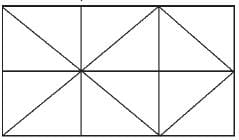Olympiad Test : Geometrical Shapes - Question 2

### ​How many parallelograms does the following figure have?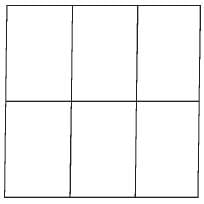Olympiad Test : Geometrical Shapes - Question 3

### ​How many triangles does the following figure have?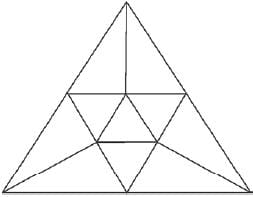Olympiad Test : Geometrical Shapes - Question 4

How many triangles and squares does the following figure have?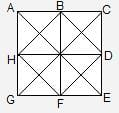Olympiad Test : Geometrical Shapes - Question 5

How many straight lines does the following figure have?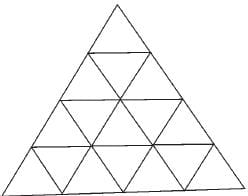Olympiad Test : Geometrical Shapes - Question 6

In each of the following questions a part of the figure is missing. Find out the light figure from the given alternatives (A), (B), (C) and (D), which will complete the figure .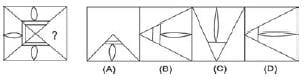Olympiad Test : Geometrical Shapes - Question 7

In each of the following questions a part of the figure is missing. Find out the light figure from the given alternatives (A), (B), (C) and (D), which will complete the figure .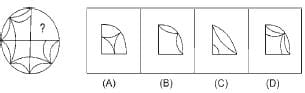Olympiad Test : Geometrical Shapes - Question 8

In each of the following questions a part of the figure is missing. Find out the light figure from the given alternatives (A), (B), (C) and (D), which will complete the figure .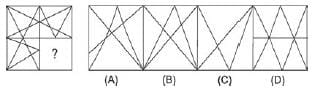Olympiad Test : Geometrical Shapes - Question 9

In each of the following questions a part of the figure is missing. Find out the light figure from the given alternatives (A), (B), (C) and (D), which will complete the figure .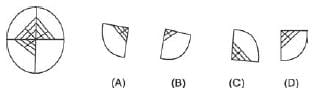Olympiad Test : Geometrical Shapes - Question 10

In each of the following questions a part of the figure is missing. Find out the light figure from the given alternatives (A), (B), (C) and (D), which will complete the figure .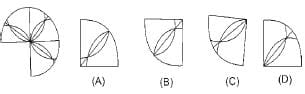Olympiad Test : Geometrical Shapes - Question 11

In each of the following questions a part of the figure is missing. Find out the light figure from the given alternatives (A), (B), (C) and (D), which will complete the figure .
Q. Which of the following dice is identical to the unfolded figure shown below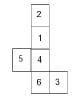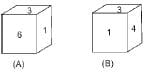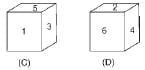Olympiad Test : Geometrical Shapes - Question 12

In each of the following questions a part of the figure is missing. Find out the light figure from the given alternatives (A), (B), (C) and (D), which will complete the figure .
Q. How many squares are in following figure?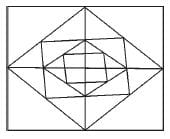Olympiad Test : Geometrical Shapes - Question 13

Select a figure from the given alternatives which when placed in the blank space of figure (X) would complete the pattern.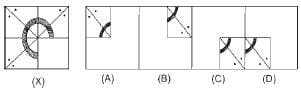Olympiad Test : Geometrical Shapes - Question 14

Select a figure from the given alternatives which when placed in the blank space of figure (X) would complete the pattern.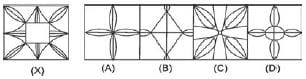Olympiad Test : Geometrical Shapes - Question 15

Select a figure from the given alternatives which when placed in the blank space of figure (X) would complete the pattern.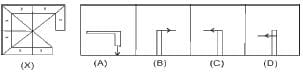Olympiad Test : Geometrical Shapes - Question 16

Select a figure from the given alternatives which when placed in the blank space of figure (X) would complete the pattern.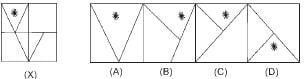Olympiad Test : Geometrical Shapes - Question 17

Select a figure from the given alternatives which when placed in the blank space of figure (X) would complete the pattern.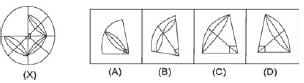Olympiad Test : Geometrical Shapes - Question 18

Select a figure from the given alternatives which when placed in the blank space of figure (X) would complete the pattern.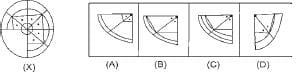Olympiad Test : Geometrical Shapes - Question 19

Select a figure from the given alternatives which when placed in the blank space of figure (X) would complete the pattern.
Q. No. of straight line in the following figure are: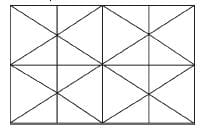Olympiad Test : Geometrical Shapes - Question 20

Select a figure from the given alternatives which when placed in the blank space of figure (X) would complete the pattern.
Q. No. of parallelograms in the following figure are: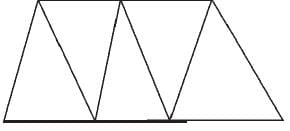## Science Olympiad for Class 5

2 videos|38 tests
 Use Code STAYHOME200 and get INR 200 additional OFF Use Coupon Code